# Conceptual Physics Notes on Chapter 33 Electric Fields

• Slides: 15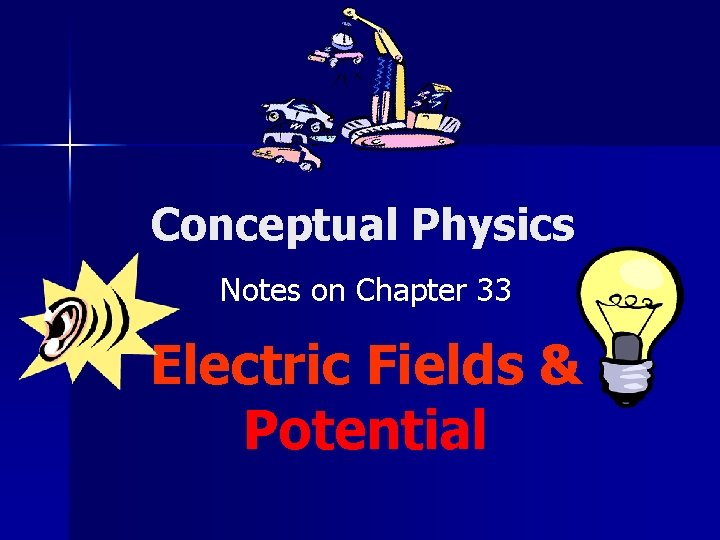Conceptual Physics Notes on Chapter 33 Electric Fields & Potential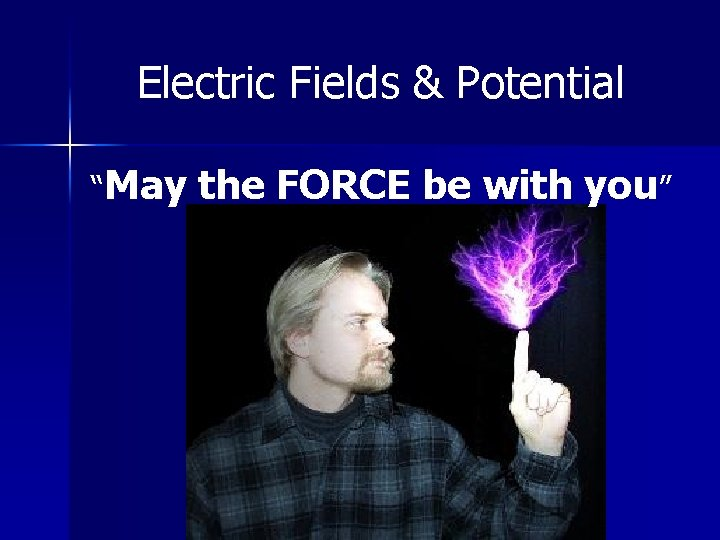Electric Fields & Potential “May the FORCE be with you”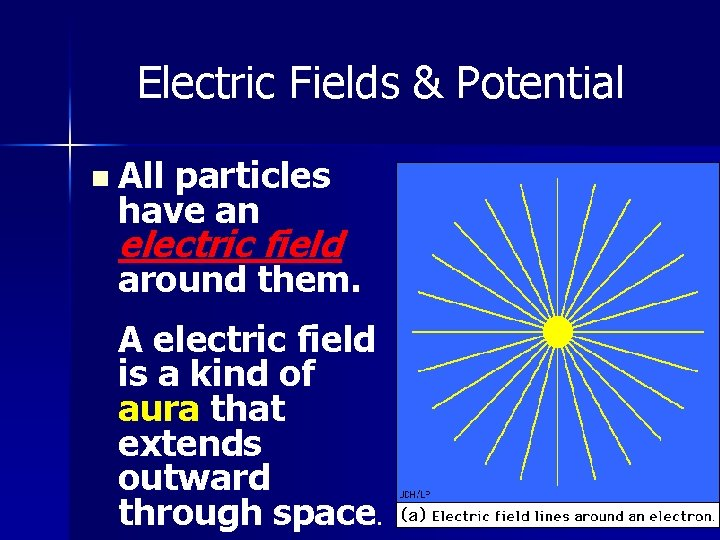Electric Fields & Potential n All particles have an electric field around them. A electric field is a kind of aura that extends outward through space.Electric Fields & Potential n To show the effects of the electric field, we use a “small test charge” (which is positive) to determine the direction and strength of the field.Electric Fields & Potential n To show the effects of the electric field, we use a “small test charge” (which is positive) to determine the direction and strength of the field.Electric Fields & Potential n Since the field has magnitude and direction it is a vector and can be represented with vectors. n These vectors are called Field lines or Lines of force n (See fig. 33. 3 pg. 519)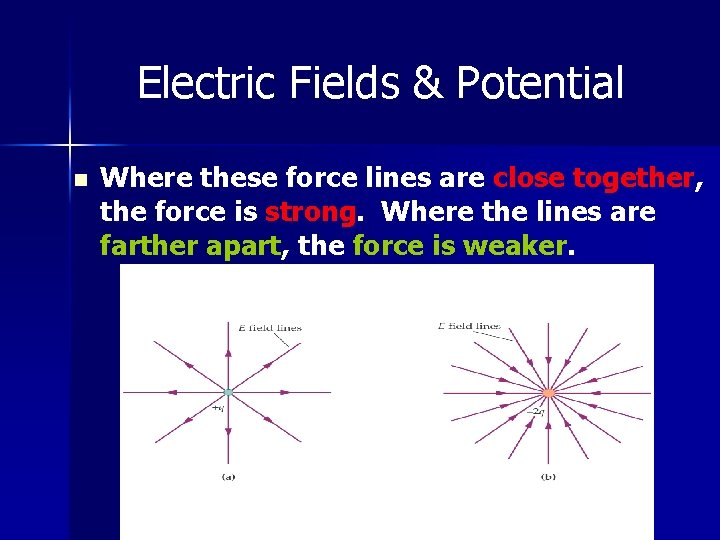Electric Fields & Potential n Where these force lines are close together, the force is strong. Where the lines are farther apart, the force is weaker.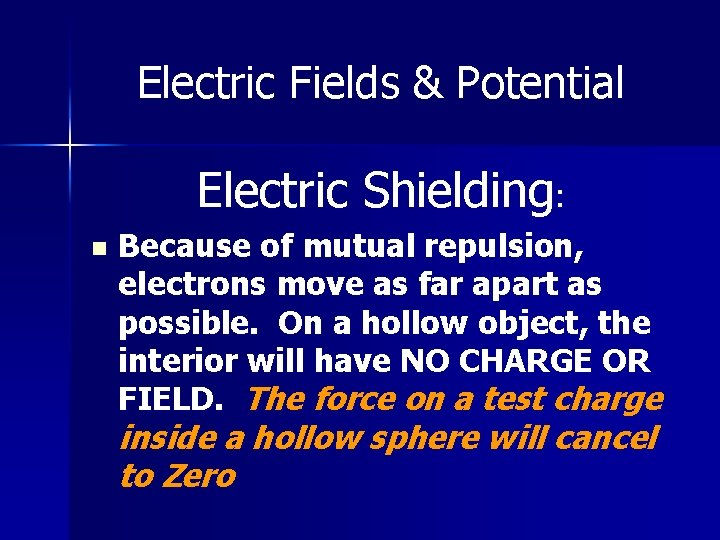Electric Fields & Potential Electric Shielding: n Because of mutual repulsion, electrons move as far apart as possible. On a hollow object, the interior will have NO CHARGE OR FIELD. The force on a test charge inside a hollow sphere will cancel to Zero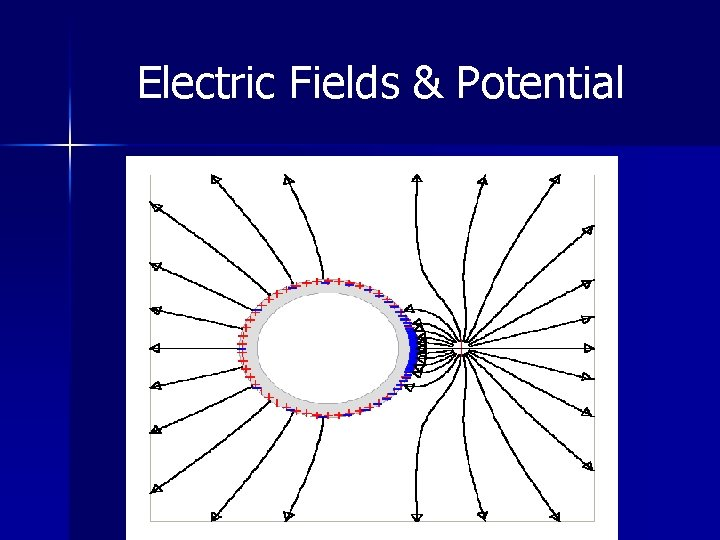Electric Fields & Potential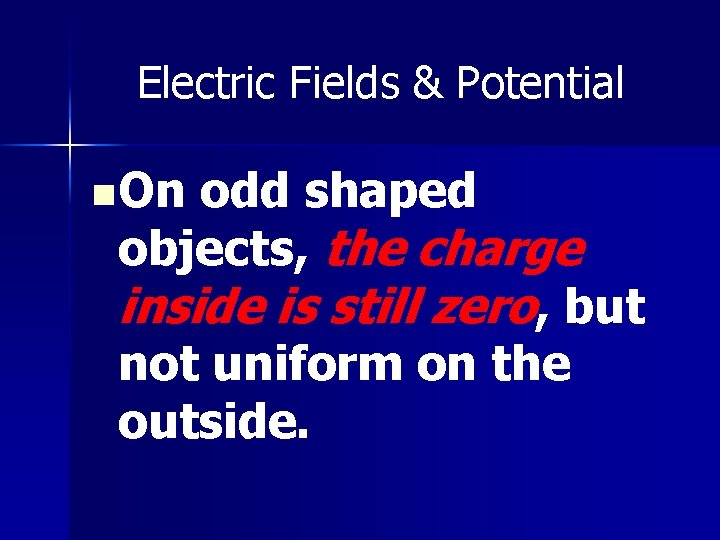Electric Fields & Potential n On odd shaped objects, the charge inside is still zero, but not uniform on the outside.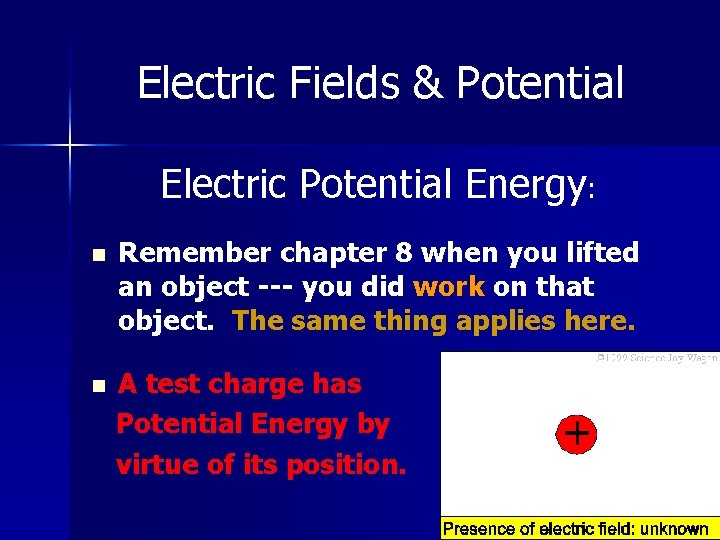Electric Fields & Potential Electric Potential Energy: n Remember chapter 8 when you lifted an object --- you did work on that object. The same thing applies here. n A test charge has Potential Energy by virtue of its position.Electric Fields & Potential n If the test charge is “let go”, the potential energy will be transferred into kinetic energy of movement.Electric Fields & Potential Electric Potential = Electric potential energy Charge 1 Volt = n 1 Joule_____ 1 Coulomb Note --- The voltage of a balloon rubbed on your head can be very high. But the electric potential energy is low because of the small amount of charge.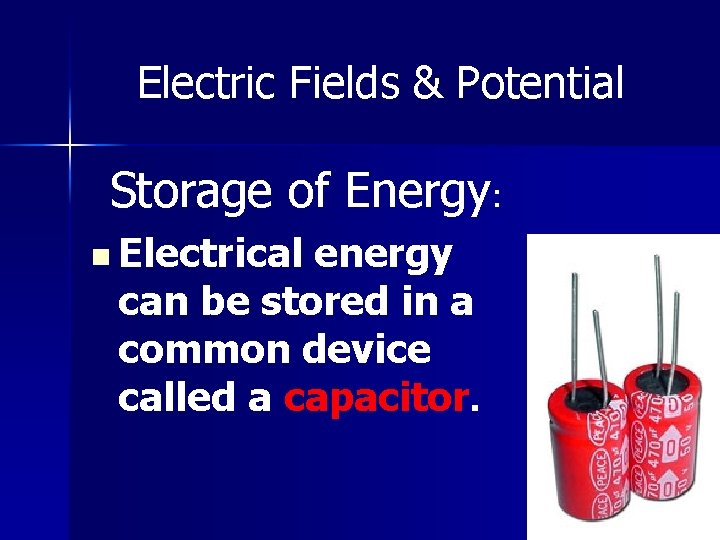Electric Fields & Potential Storage of Energy: n Electrical energy can be stored in a common device called a capacitor.Electric Fields & Potential n A capacitor is a devise that has two plates separated by a small distance and connected to a battery. n Note --- this can be a Shocking experience if you are the discharging path !